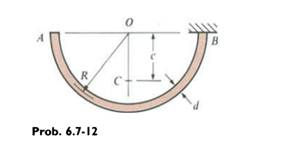### Create an Account

Already have account?

### Forgot Your Password ?

Home / Questions / A semicircular bar AB Iying in a horizontal plane is supported at B see figure The bar has...

# A semicircular bar AB Iying in a horizontal plane is supported at B see figure The bar has centerline radius R and weight q per unit of length total weight of the bar equals πqR The cross

A semicircular bar AB Iying in a horizontal plane is supported at B (see figure). The bar has centerline radius R and weight q per unit of length (total weight of the bar equals πqR). The cross section of the bar is circular with diameter d. Obtain formulas for the maximum tensile stress σmaximum compressive stress σc and maximum in-plane shear stress τmax at the top of the bar at the support due to the weight of the bar. (Note: The center of gravity of the bar is at point C, a distance c = 2R/π from the center 0.)Jun 29 2020 View more View Less

#### Answer (Solved)Subscribe To Get Solution